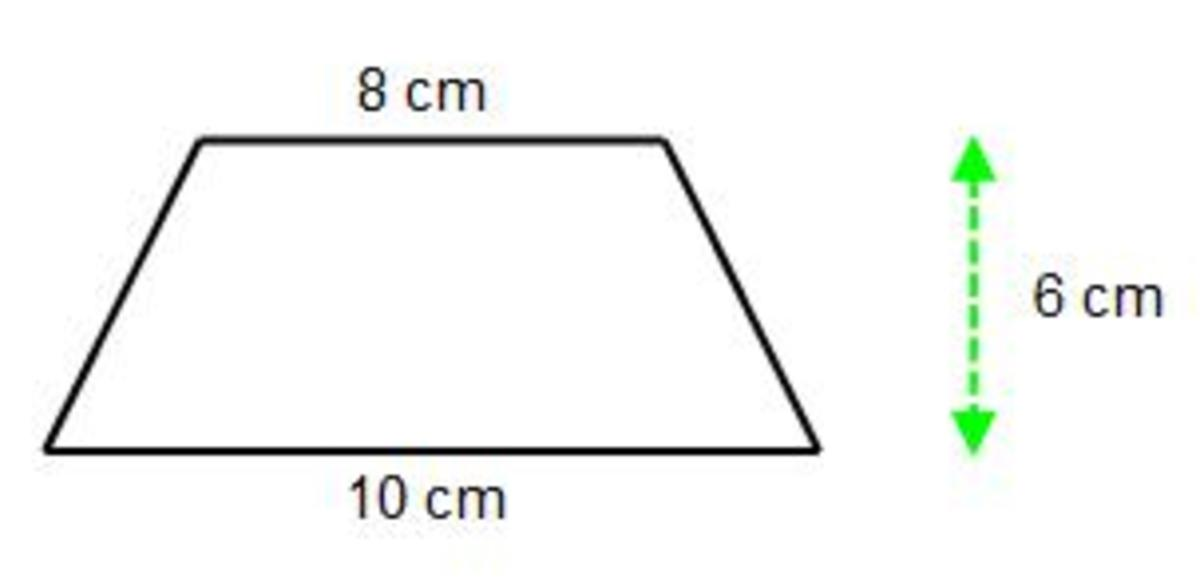# Properties of a Trapezium or Trapezoid (Math Facts)

• Author:
• Updated date:

Mark, a math enthusiast, loves writing tutorials for stumped students and those who need to brush up on their math skills.

The main property of a trapezium (as known in Great Britain) or trapezoid (as known in the United States) is that it is a 4 side shape with exactly one pair of parallel sides. The way in which the trapezium is drawn determines how many lines of symmetry it has. The trapezium at the top has one vertical line of reflectional symmetry and the trapezium at the bottom of the picture has no lines of symmetry. A trapezium doesn’t have rotational symmetry so the order of rotational symmetry is 1.

You can work out the area of a trapezium by using the formula A = ½(a+b)h. Where a and b are the lengths of the parallel sides and h is the shortest distance between the two parallel sides.

Example

Work out the area of the below trapezium.What is the area of this shape?

The two parallel sides are 8cm and 10cm. So a = 8 and b = 10. It doesn’t matter if you have these the other way around.

The shortest distance between the parallel sides is 6cm so h = 6.

All you need to do now is substitute these 3 values into the formula:

A = ½(a+b)h

A = ½ (8+10)6

A = ½ × 18 × 6 = 54 cm²

Alternatively, since the trapezium was symmetrical you could split up the trapezium into 2 triangles and a rectangle:

Area of each triangle = (6 × 1) ÷ 2 = 3cm²

Scroll to Continue

## Read More From Owlcation

Area of the rectangle = 8 × 6 = 48cm²

So the total area of the trapezium is 48 + 3 + 3 = 54 cm²

Working out the area of a trapezium is a common question on most exam papers, and you can use trapeziums to estimate the area enclosed between a curve and the coordinate axes (this is known as the trapezium rule).

So to summarise the properties of a trapezium:

a) A quadrilateral with a pair of parallel sides.

b) 0 or 1 lines of reflectional symmetry.

c) no rotational symmetry.

d) area of a trapezium A = ½(a+b)h.

## Questions & Answers

Question: What properties does a trapezium have?

Answer: A trapezium can be drawn in different ways, so the properties can vary, but it must have exactly one pair of parallel sides.

Question: What lines of symmetry does an irregular trapezium have?

Answer: It will have 0 lines of reflectional symmetry.

Question: Is a rectangle a square?

Answer: No, a rectangle is not a square.

A square has 4 equal side lengths, and a recangle has 2 pairs of equal side lengths.

Question: How many lines does a trapezoid have?

Answer: A symmetrical trapezoid will have 1 line of symmetry.

If not then the answer is 0.

Question: What properties does a rhombus have?

Answer: A rhombus has 4 equal side lengths.

The angles oppisite each other are equal.

It has 2 lines of reflectional symmetry, and order 2 rotational symmetry.

The diagonals of the rhombus bisect each other at 90 degrees.